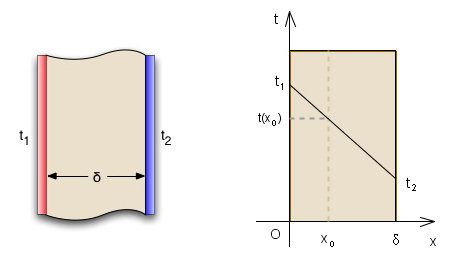•http://facebook.com/
•https://www.google.com/accounts/o8/id
•https://me.yahoo.comCOST (GBP)1.250.000

# hm plane

Computes the temperature at a given distance within a thin planar homogeneous wall.
Controller: CodeCogsContentsC++
Excel

## Hm Plane

 doublehm_plane( double x double delta double t1 double t2 )[inline]
For a planar homogeneous thin wall, the conductive heat flow per unit area is unidirectional and based on Fourier's Law it is given by:

where is the thermal conductivity of the wall (constant at any point) and .

Considering as a function of the distance within the wall, we obtain a new expression for the conductive heat flow:

Next we will make the heat flow constant at any distance within the wall:

thus obtaining the formula which gives the value of the temperature at distance within the wall:

In the left figure of the following diagram you may notice that the distance x is considered starting from the hotter side of the wall, marked in red. Also in the right figure the value of is shown for a particular value of .The example code below computes the value of for some particular input data.

### Example 1

#include <codecogs/engineering/heat_transfer/conduction/hm_plane.h>
#include <stdio.h>

int main()
{
// the distance within the wall
double x = 0.165;

// the the thickness of the wall
double delta = 0.213;

// the temperature of the entry surface for the heat flux
double t1 = 32.257;

// the temperature of the exit surface for the heat flux
double t2 = 22.421;

// display the various input values
printf("   t1 = %.3lf\n   t2 = %.3lf\ndelta = %.3lf\n", t1, t2, delta);
printf("    x = %.3lf\n\n", x);

printf("The temperature at distance %.3lf within the wall is %.5lf\n",
x, Engineering::Heat_Transfer::Conduction::hm_plane(x, delta, t1, t2));

return 0;
}
Output
t1 = 32.257
t2 = 22.421
delta = 0.213
x = 0.165

The temperature at distance 0.165 within the wall is 24.63756

### Note

The distance within the wall x must be a real positive number less than or equal to the thickness of the wall and it must be true that .

### References

Dan Stefanescu, Mircea Marinescu - "Termotehnica"

### Parameters

 x the distance within the planar wall (meters) delta the thickness of the planar wall (meters) t1 the temperature of the heat flow at the entry surface (degrees Celsius) t2 the temperature of the heat flow at the exit surface (degrees Celsius)

### Returns

The temperature at distance x within the wall (<i>degrees Celsius</i>)

### Authors

Grigore Bentea, Eduard Bentea (September 2006)
##### Source Code

Source code is available when you agree to a GP Licence or buy a Commercial Licence.

Not a member, then Register with CodeCogs. Already a Member, then Login.